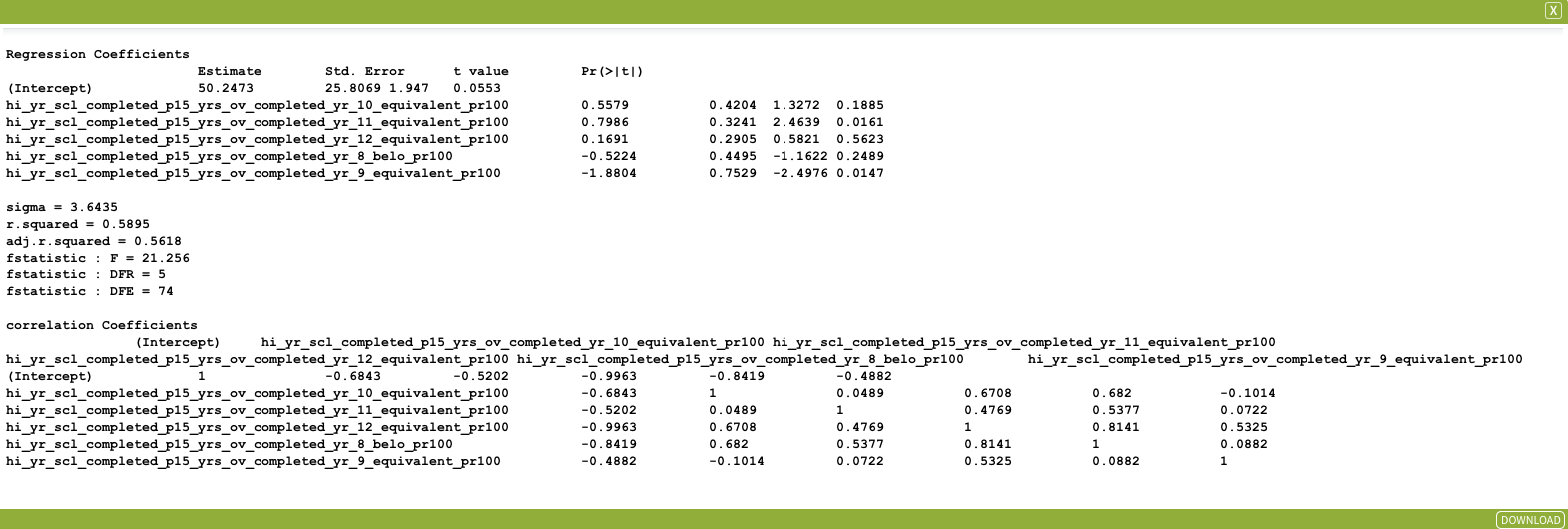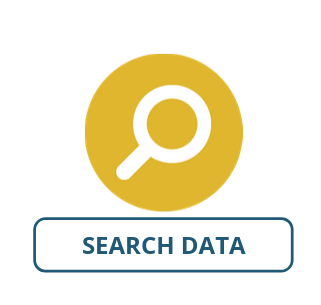Regression

Regression analysis helps estimate how a dependent variable is influenced by the change of one or more independent variables. We can use this to identify variables in our dataset that explain changes in another. This can help us understand patterns of behaviour, or changes over time.

For example, we can determine the influence of education levels on the overall participation in the labour force, or the influence a certain age group has on transport trip production.

The following formula is used in the implementation of linear regression:

$\large{\hat{y}=a+b_ix_i}$

where:
$y$ = dependent variables.
$x$ = the independent variable(s).
$\hat{y}$ = the vector of fitted values.
$a$ =  the y intercept.
$b$ = the estimate(s) of the slope.

The residual vector is $y-\hat{y}$.

SET UP

For this worked example, we will compare the levels of high school education obtained and its influence on those currently in the labour force on LGA regions in Victoria.

Select the state of Victoria as your area.

Select ABS – Data by Region – Education & Employment (LGA) 2011-2017 as your dataset with the following attributes:

• Year (with Filter Value: 2011).
• Highest Year Of School Completed – Persons Ages 15 Years And Over Completed Year 8 Or Below %.
• Highest Year Of School Completed – Persons Ages 15 Years And Over Completed Year 9 Or Equivalent %.
• Highest Year Of School Completed – Persons Ages 15 Years And Over Completed Year 10 Or Equivalent %.
• Highest Year Of School Completed – Persons Ages 15 Years And Over Completed Year 11 Or Equivalent %.
• Highest Year Of School Completed – Persons Ages 15 Years And Over Completed Year 12 Or Equivalent %.
• Labour Force Statistics Participation Rate %.
• LGA Code.
• LGA Name.

Inputs

Once you have set up your data, open the Regression tool (Tools → Statistical Analysis → Regression). The input fields are as follows:

• Dataset Input: The dataset containing the variables you would like to test. SelectABS – Data by Region – Education & Employment (LGA) 2011-2017.
• Dependent Variable: The variable we would like to test. Select: Labour Force Statistics Participation Rate %.
• Independent Variable(s): The independent variables that we would like to test against.
• Select: Highest Year Of School Completed – Persons Ages 15 Years And Over Completed Year 8 Or Below %.
• Select: Highest Year Of School Completed – Persons Ages 15 Years And Over Completed Year 9 Or Equivalent %.
• Select: Highest Year Of School Completed – Persons Ages 15 Years And Over Completed Year 10 Or Equivalent %
• Select: Highest Year Of School Completed – Persons Ages 15 Years And Over Completed Year 11 Or Equivalent %.
• Select: Highest Year Of School Completed – Persons Ages 15 Years And Over Completed Year 12 Or Equivalent %.

The input parameters are summarised in the image below, once complete click Run Tool.

Outputs

Once the tool has run, click the Display button on the pop-up dialogue box that appears. This will open a window with the outputs of your regression analysis, which should look like the image below. This output displays a matrix of regression coefficients, the parameters of the analysis, and a matrix of correlation coefficients.By looking at the p-value in the Pr(>|t|) column, we can see that focusing on the percentage of those who have completed year 9 and 11 could be beneficial to our analysis as they have values less than 0.05. By looking at the coefficients of these variables, we see that the population with a maximum of a year 9 education decreases the value of active participation in the labour market, where as those with a year 11 education increases it. Looking further into the output parameters allows us to identify to what extent these variables may help us in a particular study.

The outputs of the regression analysis are explained below:

• Intercept: The slope-intercept.
• Estimate(s): The coefficient estimate for each variable.
• Std. Error: The average distance that observed values are from the regression line.
• T-value: The estimated value divided by its estimated standard error.
• Pr(>|t|): The probability for testing the hypothesis.
• Sigma: Standard deviation.
• R.squared: R2 Co-efficient of determination illustrating the amount of variation in the dependent variable explained by variation in the independent variables.
• F-statistic:
• F value, illustrating the ratio of two measures of variability.
• DFR illustrating the degrees of freedom for regression.
• DFE illustrating the degree of freedom for error.
• The correlation coefficient matrix contains the measurements of how two variables are related.

Buechler, S. (2007). Statistical Models in R – Some Examples. University of Notre Dame.
Ferrari, D., & Head, T. (2010). Regression in R Part I: Simple Linear Regression. UCLA Department of Statistics-Statistical Consulting Center.
Residual sum of squares. (2019). In Wikipedia. http://en.wikipedia.org/wiki/Residual_sum_of_squares

Looking for Spatial Data?

You can browse the AURIN Data Discovery:How can you Create Impact?